## A sample of 7.55 g of He has a volume of 5,520 mL and a temperature of 123°C. What is its pressure in torr?

Question

A sample of 7.55 g of He has a volume of 5,520 mL and a temperature of 123°C. What is its pressure in torr?

in progress 0
2 months 2021-08-22T18:22:28+00:00 1 Answers 3 views 0

1. Answer: The pressure is 8451.2 torr.

Explanation:

Given: Mass of He = 7.55 g

Volume = 5520 mL

Convert mL into L as follows.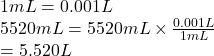Temperature =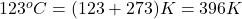Now, moles of He (molar mass = 4.00 g/mol) are as follows.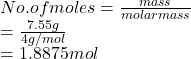Formula used to calculate pressure is as follows.

PV = nRT

where,

P = pressure

V = volume

n = no. of moles

R = gas constant = 0.0821 L atm/mol K

T = temperature

Substitute the values into above formula as follows.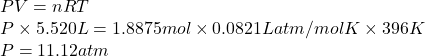Convert atm into torr as follows.

1 atm = 760 torr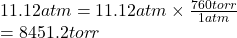Thus, we can conclude that the pressure is 8451.2 torr.